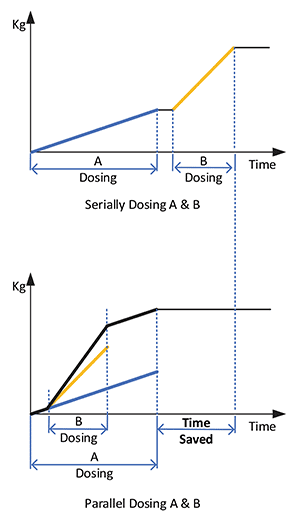This article shows a method where on vessels with load cells, and no other means of quantifying the material additions, load cells and a control system can be used to perform material additions. (See Figure 1, above.) The required amount setpoint is specified in addition to the required tolerance.

The material addition process starts by capturing a reference weight, the required equipment is commanded to start the required material transfer, the weight is monitored until the required cutoff amount is achieved, and the system then commands the equipment to stop transferring material. After the material transfer completes, the actual amount dosed is calculated and verified to be within the required tolerance.

Cutoff setpoint (CSP) = Reference weight + Setpoint (SP) – Spill (S)

If more than one material is required this process is repeated for all material, these material additions occur in series since there is only one instrument to quantify the amount added. (See Figure 2, above.)

Some processes can benefit if they could add more than one material at the same time. This improvement is normally achieved by adding flowmeters or pre-weigh equipment to the material streams, but these require investments that the process owners may not be ready to make.

The following is an approach that allows the addition of multiple streams of materials to a vessel whose only mean of totalizing materials is via the existent (single set) load cells and additional controller code. Material dosing can continue to be added in series or in parallel; this method is based on basic Calculus principles such as derivatives and integrals, which can be executed with standard controller instructions.

The method consists of the following actions:

Determine the flow rate of material entering the vessel; this is obtained by taking the first derivative of the weight of the tank.

Dwt/Dt = T_Flow

The T_Flow flow rate is integrated to calculate the total amount of material calculated by the controller logic; yet, this should be the same amount gained by the tank since the Mathematical Integral of the T_Flow was started. This comparison confirms that the equation is valid.Figure 3: Serially dosing ingredient A, then B, causes a period of time when neither ingredient is dosed. Parallel dosing removes the time gap, providing dosing time savings. Source: John Parraga

Based on actual process input, an equation is developed to describe the theoretical behavior of the flow (TH_Flow); this TH_Flow is integrated and compared to the actual totalized amount of the load cells. If the theoretical equation is within tolerance of the actual amount this means that the calculated equation is a good fit. These calculations are compared and analyzed to quantify the accuracy of the TH_Flow equation.

At this point, it can be inferred that the integral of the TH_Flow is a good representation of the flow of one of the streams required, and it’s integral a good representation of the amount added.

At this point, a second stream can be started (the one that requires less dosing time based on historical feed rates and the required amount setpoints). Upon starting the second stream, the additional flow entering the vessel can be calculated based on the difference between the Total flow (T_Flow) and the Theoretical Flow (TH_Flow), (2_Flow). This new flow rate can be integrated to determine the amount of material of the second stream entering the vessel.

Individual dosing totalizers are used for each material being dosed; each of them will determine when the cutoff for their steam should occur.

Based on process flow variabilities, additional confidence can be obtained by pausing material feeds and recalculation the equations. This method is best suited for material whose flow variability is not randomized throughout the feed, but this can be determined ahead of time, and the system can automatically pause a stream if this is detected.

The addition of multiple materials in parallel (Figure 3) will reduce the batch cycle by reducing the amount of time required to load the required ingredients. This method is a low risk, simple-to-prove additional controller code that can be used to optimize the process overall batch effectiveness.